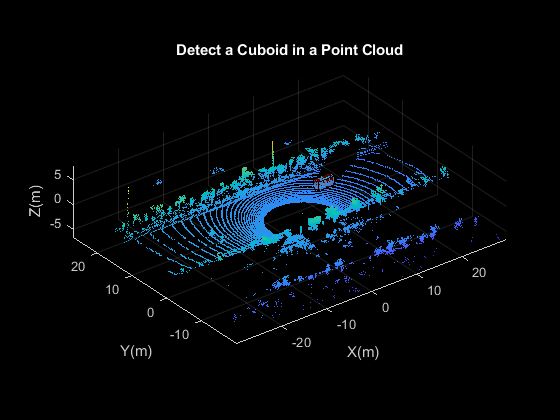# plot

Plot cuboid model

## Syntax

``plot(model)``
``plot(model,'Parent',ax)``
``H = plot(___)``

## Description

example

````plot(model)` plots a cuboid model within the axes limits of the current figure.```
````plot(model,'Parent',ax)` plots a cuboid model on a specified output axes.```
````H = plot(___)` additionally returns the cuboid model plot (figure) as a `patch` object.```

## Examples

collapse all

Detect a cuboid in a point cloud using `pcfitcuboid` function. The function stores the cuboid parameters as a `cuboidModel` object.

Read point cloud data into the workspace.

`ptCloud = pcread('highwayScene.pcd');`

Search the point cloud within a specified region of interest (ROI). Create a point cloud of only the detected points.

```roi = [-30 30 -20 30 -8 13]; in = findPointsInROI(ptCloud,roi); ptCloudIn = select(ptCloud,in);```

Plot the point cloud of detected points.

``` figure pcshow(ptCloudIn.Location) xlabel('X(m)') ylabel('Y(m)') zlabel('Z(m)') title('Detected Points in ROI')```Find the indices of the points in a specified ROI within the point cloud.

```roi = [9.6 13.8 7.9 9.3 -2.5 3]; sampleIndices = findPointsInROI(ptCloudIn,roi);```

Fit a cuboid to the selected set of points in the point cloud.

``` model = pcfitcuboid(ptCloudIn,sampleIndices); figure pcshow(ptCloudIn.Location) xlabel('X(m)') ylabel('Y(m)') zlabel('Z(m)') title('Detect a Cuboid in a Point Cloud')```

Plot the cuboid box in the point cloud.

```hold on plot(model)```Display the internal properties of the `cuboidModel` object.

`model`
```model = cuboidModel with properties: Parameters: [11.4873 8.5997 -1.6138 3.6713 1.3220 1.7576 0 0 0.9999] Center: [11.4873 8.5997 -1.6138] Dimensions: [3.6713 1.3220 1.7576] Orientation: [0 0 0.9999] ```

## Input Arguments

collapse all

Cuboid model, specified as a `cuboidModel` object.

Output axes, specified as an `Axes` object, on which to display the cuboid model. For a list of properties, see Axes Properties.

## Output Arguments

collapse all

Patch object, returned as a `patch` object.

### Objects

Introduced in R2020b

## SupportGet trial now